Mersenne prime

This article defines a property that can be evaluated for a prime number. In other words, every prime number either satisfies this property or does not satisfy this property.
View other properties of prime numbers | View other properties of natural numbers

Definition

A Mersenne prime is a Mersenne number that is also a prime number. In other words, it is a number of the form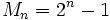that is prime, whereis a natural number.

It turns out that if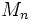is prime, thenitself is also prime, though the converse is not true (the smallest counterexample is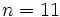, because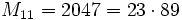).

Occurrence

Initial examples

The Mersenne numbers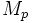are prime for, with the corresponding primesbeing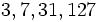. Them smallest primefor which the Mersenne numberis not prime is:.

Infinitude conjecture

Further information: Infinitude conjecture for Mersenne primes

It is conjectured that there are infinitely many Mersenne primes.